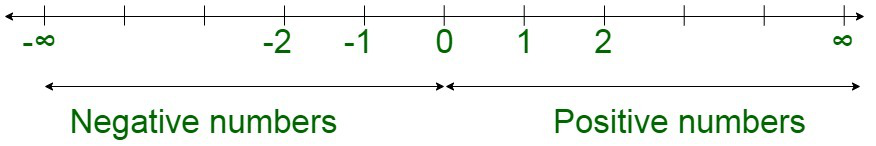GeeksforGeeks App
Open AppBrowser
Continue

# What is the difference between positive and negative numbers?

Algebra is a special branch of mathematics that is used to deal with the arithmetic operations, such as addition, subtraction, multiplication, or division, and the associated symbols, also known as variables. The variables are not fixed and change their value. Some of the examples of variables are x,y, and z.

Zero

Zero holds a unique distinction of holding neither positive nor negative value. Zero segregates the positive numbers from the negative ones. The negative numbers lie on the left of the number line, indicated as, –4, –3, –2, –1, 0, whereas positive numbers are, 1, 2, 3, 4.

Positive Numbers

Positive Numbers are the integral numbers that are greater than equal to zero. Positive numbers are infinite in number. In other words, any number above zero is counted as a positive number. Positive numbers lie on the right of the number line. For instance, savings are positive. Positive numbers can include rational numbers, irrational numbers, or complex numbers. The positive numbers increase in magnitude as they move farther from the number line.

Negative Numbers

Negative Numbers are the integral numbers that are less than zero. In other words, any number below zero is counted as a negative number. Negative numbers lie on the left of the number line. For instance, loans are negative. The negative numbers can include rational numbers, irrational numbers, or complex numbers. The negative numbers decrease in magnitude as they move farther from the number line.

The following diagram can be used to illustrate the positive and negative numbers on a number line:The following table illustrates the differences between the negative and positive numbers respectively:

Properties of positive and negative numbers

• Every positive number has an equal and opposite negative counterpart.
• Every negative number can be considered as a negation of a positive number.

Conversion of positive and negative numbers

The positive numbers can be converted to negative numbers by multiplying by the value of -1, similarly vice versa. This multiplication is used to achieve negation of the corresponding counterpart.

For instance, converting 5 to a negative number can be done by:

5 × (-1) = -5 , which is a negative number

Also, -8 can be converted to a positive number can be done by:

-8 × (-1) = 8, which is a positive number

### Sample Problems

Question 1: Are positive and negative numbers equal in value?

Solution:

Yes, since every positive number has an equal and opposite negative value. Therefore, positive and negative numbers are equal in values.

Question 2. State some applications of negative numbers.

Solution:

• Temperature – It represents expressing the hotness and coldness. A positive temperature is indicative of hot temperature while a negative temperature indicates cold weather.
• Money – Negative money represents credit in the banking
• Elevator/Lift – The floors corresponding to below the ground floor such as the basement or parking are marked with negative numbers (e.g., -1,-2,-3.)

Question 3. Which of the following numbers are negative and positive numbers?

3/-5, -1/2, 10/3, -4/-12, √2, 13/√-5, -2/-9, 5/6

Solution:

Here,

Positive Numbers

10/3, -4/-12, √2, -2/-9, 5/6

Negative Numbers

3/-5, -1/2, 13/√-5

Question 4. List any 5 Positive rational numbers?

Solution:

Rational numbers: It is represented in the form of p/q, where q≠0.

Here the 5 positive rational numbers will be

3/5, -1/-2, 4/8, 9/5, -3/-10

My Personal Notes arrow_drop_up
Related Tutorials